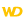HelpWLanguageWLanguage functionsStandard functionsNumeric values management functionsFinancial functionsFinCurrentVal (Example)Current value of an investmentFinCurrentVal (Example)FinCurrentValFinDecreasingRedemption (Example)FinDecreasingRedemptionFinError (Example)FinErrorFinFutureVal (Example)FinFutureValFinInterestRate (Example)FinInterestRateFinLinearRedemption (Example)FinLinearRedemptionFinNetCurrentVal (Example)FinNetCurrentValFinPaymentNb (Example)FinPaymentNbFinPeriodInterest (Example)FinPeriodInterestFinRedemption (Example)FinRedemptionFinRepayVal (Example)FinRepayValFinancial constantsFinancial functions
 Current value of an investment FinCurrentVal (Example)See the associated help page Current value of an investmentThe following code is used to calculate the current value of an investment. The payment value, the future value, the number of periods and the interest rate are entered by the user in edit controls (EDT_PaymentValue, EDT_FutureValue, EDT_NbPeriod, EDT_Rate).We assume that the payments must be made at the end of the period (False constant). // -- Click code on BTN_CurrentValue// Declare the variableResCurrentValue is real// Calculate the current valueResCurrentValue = FinCurrentVal(-EDT_PaymentValue, EDT_FutureValue, EDT_NbPeriod, EDT_Rate, False)IF FinError() = 0 THEN Info("The current value is: " + ResCurrentValue)ELSE // Display the error message Error(ErrorInfo(errMessage))END Minimum version required Version 9 This page is also available for…